# Related Angles

Related Angles are the pair of angles that are related to each other. For each pair of related angles, there is a particular name given to them. These angles are related to each other based on some specific conditions.

Two angles are said to be related if they satisfy certain criteria. For example, when the transversal cuts the parallel lines, then there are certain angles that are formed such as corresponding angles, alternate interior angles, vertical angles, etc. Thus, these angles are related to each other with respect to the condition of transversal and parallel lines.

## Types of Related Angles

The five types of related angles are:

Let us discuss all these related angles and their corresponding conditions by which they are related to each other.

### Complementary Angles

The pair of angles that results in 90 degrees, when added together, are called complementary angles. Here, one angle is the complement of another angle. Hence, the complementary angles are related to each other by 90 degrees.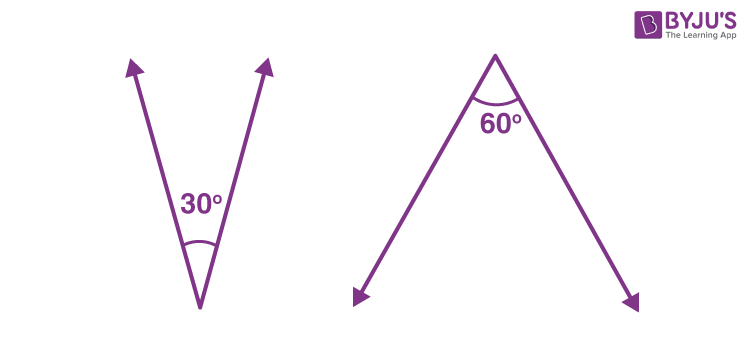In simple words, when the sum of two angles is equal to 90°, then they are known as complementary angles. The examples of complementary angles are:

• 30° and 90° (30° angle is the complement of 90°angle)
• 20° and 70° (70°angle is the complement of 70° angle)
• 40° and 50° (40° angle is the complement of 50° angle)
 Facts: Two acute angles are always complement to each other (Since, acute angle < 90°) Two obtuse angles cannot be complement to each other (Since, obtuse angle > 90°) Two right angles cannot be complement to each other (Since right angle = 90°)

### Supplementary Angles

When the sum of any two angles is equal to 180 degrees, then such pairs of angles are called supplementary angles. In this case, one angle is said to be a supplement of the other. Thus, the supplementary angles are related to each other by 180 degrees.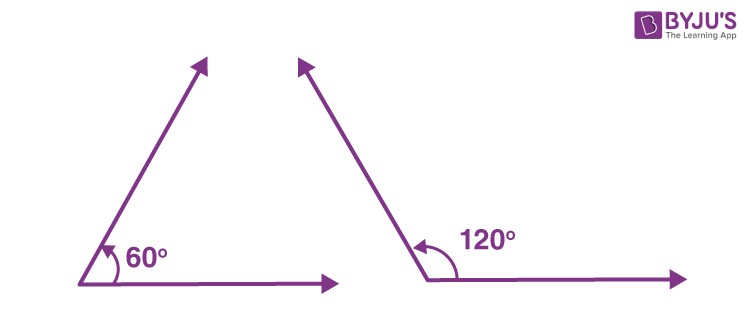The examples of supplementary angles are:

• 60° and 120° (since 60° angle is the supplement of 120° angle)
• 100° and 80° (since 100° angle is the supplement of 80° angle)
• 90° and 90° (since 90° angle is the supplement of another 90° angle)
 Facts: Two obtuse angles cannot be supplementary Two acute angles cannot be supplementary Two right angles are always supplementary to each other

Two angles are said to be adjacent angles when they have a common vertex and a common arm. There shall be no common interior points between them.

The adjacent angles have common vertex, common arm but the non-common arms are on either side of the common arm. See the below figure to understand the difference between adjacent and non-adjacent angles.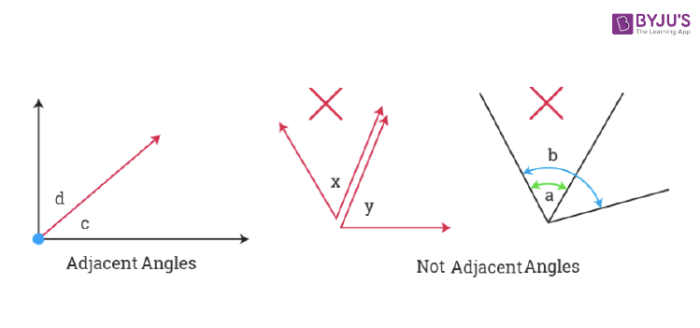In the above figure, angle c and angle d are adjacent angles.

Angle x and angle y are non-adjacent angles

Also, although angle a and angle b have common vertices, they are still non-adjacent angles.

### Linear Pair

We have learned about the adjacent angles in the above section. Now, if two adjacent angles together form a straight angle or 180 degree angle, then they are said to be a linear pair.

The noncommon sides of the linear pair of angles are opposite rays. Also, the angles in a linear pair are supplementary to each other. Thus, linear pairs are related angles.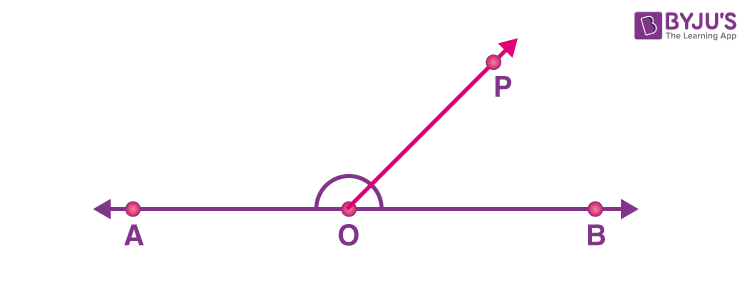The examples of linear pair of angles are:

• 60° and 120° (with one arm and vertex common to each other)
• 100° and 80° (with one arm and vertex common to each other)
• 90° and 90° (with one arm and vertex common to each other)
 Facts: Two acute angles cannot form linear pair (because the resulting angle will be less than 180 degrees) Two obtuse angles cannot form linear pair (because the resulting angle will be more than 180 degrees) Two right angles when combined together with a common arm and a common vertex, always form a linear pair.

### Vertically Opposite Angles

When two lines intersect or meet each other at a single point, then the vertically opposite angles formed are equal.

To be noted, there are four angles formed when two lines cut each other at a point. Thus, vertical angles that are opposite to each other at that point are equal. Therefore, such angles are related to each other in these terms. See the figure below, to understand better.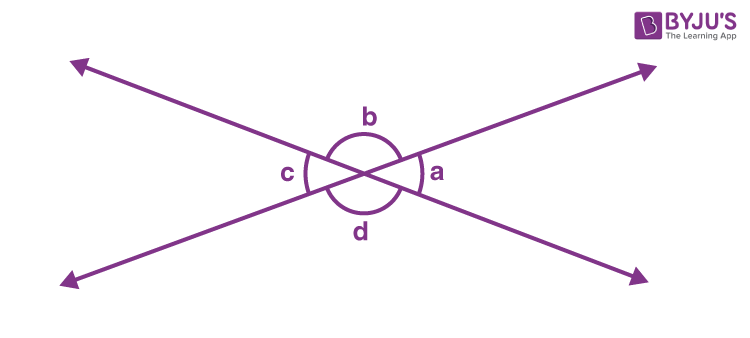In the above figure, four angles are formed by the intersection of two lines, such that:

∠a = ∠c

∠b = ∠d

## Solved Examples

Q.1: What is the complement of 33°?

Solution: Let the required angle be x

Thus,

X + 33° = 90° [Complementary angles condition]

X = 90° – 33°

X = 57°

Therefore, the complement of 33° is 57°.

Q.2: What is the supplement of angle equal to 105°?

Solution: Let the required angle be x.

So,

X + 105° = 180° [Supplementary angles condition]

X = 180° – 105°

X =75°

Therefore, 75° and 105° are supplementary angles.

### Practice Questions

1. X and Y are complementary angles. If X = 23°, then find the value of Y.
2. A and B are supplementary angles.If A = 88°, then find the value of B.
3. An angle is greater than 90º. Is its supplementary angle greater than 90º or equal to 90º or less than 90º?
4. Give two examples of adjacent angles.
5. Give two examples of vertically opposite angles.